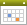##Write a shell script to Accept number and check the number is even or odd, finds the length of the number, sum of the digits in the number.

clear
echo "Enter Number : "

count=0
total=0

if [ `expr \$no % 2` -eq 0 ]
then
echo "NUMBER IS EVEN"
else
echo "NUMBER IS ODD"
fi

while [ \$no -ne 0 ]
do
a=`expr \$no % 10`
no=`expr \$no / 10`
total=`expr \$total + \$a`
count=`expr \$count + 1`
done

echo "Sum of All Digit : \$total"
echo "Total No. of Digit : \$count"